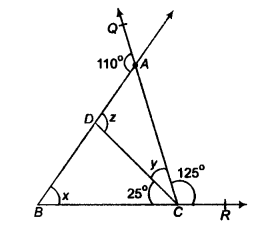# Given figure, if ∠BCD = 25°, ∠BAQ =110° and ∠ACR =125°,then find the values of x, y and z

In the given figure, if ∠BCD = 25°, ∠BAQ =110° and ∠ACR = 125°, then find the values of x, y and z.Since, BCR is a straight line.
∴ ∠BCD + ∠DCA + ∠ACR = 180°
=>25° + y + 125° =180°
=>y = 180° -150°
=>y = 30…(i)
[linear pair axiom]